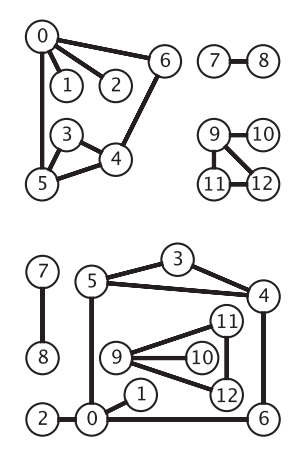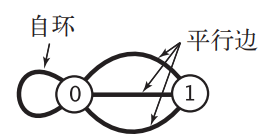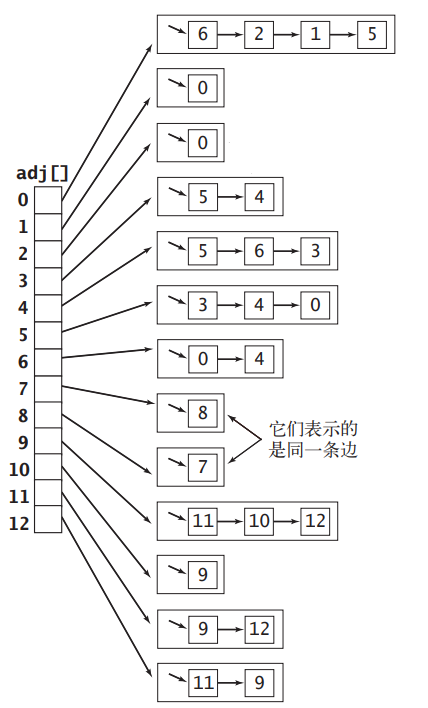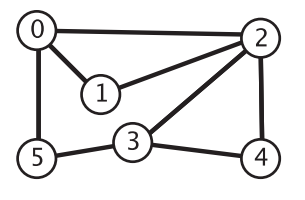# 如何在 Java 中实现无向图# 基本概念

## 无向图的定义# 无向图的 API

package com.zhiyiyo.graph;

/**
* 无向图
*/
public interface Graph {
/**
* 返回图中的顶点数
*/
int V();

/**
* 返回图中的边数
*/
int E();

/**
* 向图中添加一条边
* @param v 顶点 v
* @param w 顶点 w
*/

/**
* 返回所有相邻顶点
* @param v 顶点 v
* @return 所有相邻顶点
*/
}


# 无向图的实现方式

## 邻接矩阵

a_{ij} = \left\{ \begin{aligned} 1& \quad (v_i,v_j)\in E\\ 0& \quad 其他 \end{aligned} \right.## 邻接表数组package com.zhiyiyo.graph;

/**
* 使用邻接表实现的无向图
*/
public class LinkGraph implements Graph {
private final int V;
private int E;

this.V = V;
for (int i = 0; i < V; i++) {
}
}

@Override
public int V() {
return V;
}

@Override
public int E() {
return E;
}

@Override
public void addEdge(int v, int w) {
E++;
}

@Override
}
}


package com.zhiyiyo.collection.stack;

import java.util.EmptyStackException;
import java.util.Iterator;

/**
* 使用链表实现的堆栈
*/
private int N;
private Node first;

public void push(T item) {
first = new Node(item, first);
N++;
}

public T pop() throws EmptyStackException {
if (N == 0) {
throw new EmptyStackException();
}

T item = first.item;
first = first.next;
N--;
return item;
}

public int size() {
return N;
}

public boolean isEmpty() {
return N == 0;
}

public Iterator<T> iterator() {
return new ReverseIterator();
}

private class Node {
T item;
Node next;

public Node() {
}

public Node(T item, Node next) {
this.item = item;
this.next = next;
}
}

private class ReverseIterator implements Iterator<T> {
private Node node = first;

@Override
public boolean hasNext() {
return node != null;
}

@Override
public T next() {
T item = node.item;
node = node.next;
return item;
}

@Override
public void remove() {
}
}
}


# 无向图的遍历package com.zhiyiyo.graph;

public interface Search {
/**
* 起点 s 和 顶点 v 之间是否连通
* @param v 顶点 v
* @return 是否连通
*/
boolean connected(int v);

/**
* 返回与顶点 s 相连通的顶点个数（包括 s）
*/
int count();

/**
* 是否存在从起点 s 到顶点 v 的路径
* @param v 顶点 v
* @return 是否存在路径
*/
boolean hasPathTo(int v);

/**
* 从起点 s 到顶点 v 的路径，不存在则返回 null
* @param v 顶点 v
* @return 路径
*/
Iterable<Integer> pathTo(int v);
}


## 深度优先搜索

package com.zhiyiyo.graph;

import com.zhiyiyo.collection.stack.Stack;

public class DepthFirstSearch implements Search {
private boolean[] marked;
private int[] edgeTo;
private Graph graph;
private int s;
private int N;

public DepthFirstSearch(Graph graph, int s) {
this.graph = graph;
this.s = s;
marked = new boolean[graph.V()];
edgeTo = new int[graph.V()];
dfs();
}

/**
* 递归实现的深度优先搜索
*
* @param v 顶点 v
*/
private void dfs(int v) {
marked[v] = true;
N++;
for (int i : graph.adj(v)) {
if (!marked[i]) {
edgeTo[i] = v;
dfs(i);
}
}
}

/**
* 堆栈实现的深度优先搜索
*/
private void dfs() {
vertexes.push(s);
marked[s] = true;

while (!vertexes.isEmpty()) {
Integer v = vertexes.pop();
N++;

// 将所有相邻顶点加到堆栈中
for (Integer i : graph.adj(v)) {
if (!marked[i]) {
edgeTo[i] = v;
marked[i] = true;
vertexes.push(i);
}
}
}
}

@Override
public boolean connected(int v) {
return marked[v];
}

@Override
public int count() {
return N;
}

@Override
public boolean hasPathTo(int v) {
return connected(v);
}

@Override
public Iterable<Integer> pathTo(int v) {
if (!hasPathTo(v)) return null;

int vertex = v;
while (vertex != s) {
path.push(vertex);
vertex = edgeTo[vertex];
}

path.push(s);
return path;
}
}



## 广度优先搜索

package com.zhiyiyo.graph;

public class BreadthFirstSearch implements Search {
private boolean[] marked;
private int[] edgeTo;
private Graph graph;
private int s;
private int N;

public BreadthFirstSearch(Graph graph, int s) {
this.graph = graph;
this.s = s;
marked = new boolean[graph.V()];
edgeTo = new int[graph.V()];
bfs();
}

private void bfs() {
marked[s] = true;
queue.enqueue(s);

while (!queue.isEmpty()) {
int v = queue.dequeue();
N++;

for (Integer i : graph.adj(v)) {
if (!marked[i]) {
edgeTo[i] = v;
marked[i] = true;
queue.enqueue(i);
}
}
}
}
}



package com.zhiyiyo.collection.queue;

import java.util.EmptyStackException;

private int N;
private Node first;
private Node last;

public void enqueue(T item) {
Node node = new Node(item, null);
if (++N == 1) {
first = node;
} else {
last.next = node;
}
last = node;
}

public T dequeue() throws EmptyStackException {
if (N == 0) {
throw new EmptyStackException();
}

T item = first.item;
first = first.next;
if (--N == 0) {
last = null;
}
return item;
}

public int size() {
return N;
}

public boolean isEmpty() {
return N == 0;
}

private class Node {
T item;
Node next;

public Node() {
}

public Node(T item, Node next) {
this.item = item;
this.next = next;
}
}
}



# 后记

2 total views,  1 views today